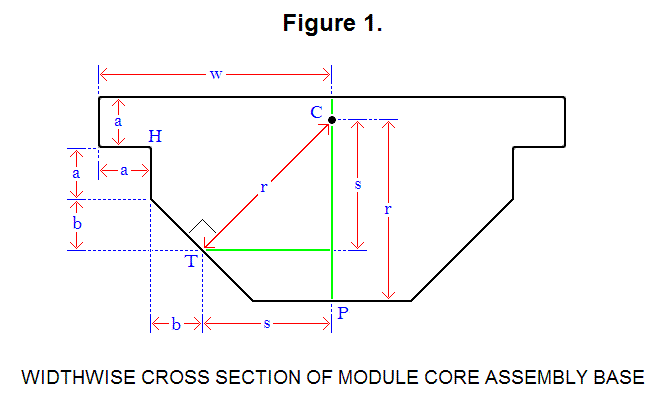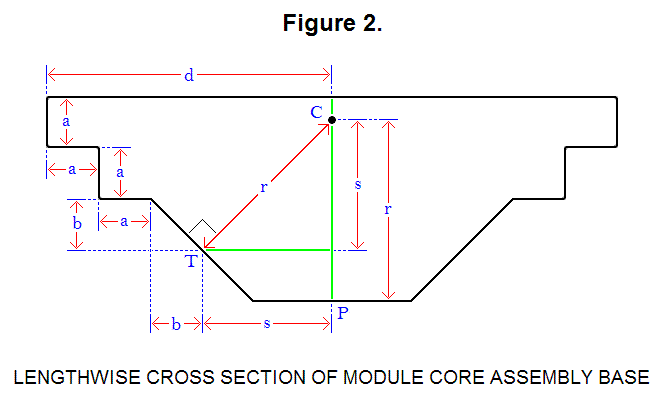# OPTIMAL RATIO CALCULATION FOR MODULE CORE ASSEMBLY BASE COMPONENT

Six assemblies, and an optional power cube, form a module; each assembly is made up of several distinct components: a telescoping leg, one base that connects directly to the telescoping leg (the connecting plate), a module core base, and an intermediate component that connects the telescoping leg to the module core base. To maximize the space for the intermediate component of the assembly that connects the telescoping leg to the assembly’s module core connector base, there are optimal ratios for the lengths of the sides of the part of the assembly that connects to 4 other assemblies and the power cell (the module core assembly base), to form a module, and they can be calculated. Figure 1, below, is a diagram that shows a widthwise cross section of this component of the assembly.Optimization is accomplished by setting the length of line segment CP to line segment CT and line segment CH, which are all equal to length r. For this diagram, the following equations are derived or set:

√2s = r                                                         (Equation 1)
w = a + b + s     →     w – a = b + s            (Equation 2)
s + a/2 = b + 2a     →      s = b + 3a/2      (Equation 3)
CP = CT = CH = r                                        (Equation 4)
(a/2)2 + (b + s)2 = r2                                  (Equation 5)

he ratios can be boiled down to a specific ratio k between 2 lengths, a and w (a ∝ w → ka = w); to solve for the constant of proportionality k, solve for a and w:

Plug equation 3 into equation 2:

w – a = 2b + 3a/2     →     b = w/2 – 5a/4      (Equation 6)

Plug equation 3 into equation 1:

√2(b + 3a/2) = r      (Equation 7)

Plug equation 2 into equation 5:

(a/2)2 + (w – a)2 = r2      (Equation 8)

Plug equation 7 into equation 8:

(a/2)2 + (w – a)2 = 2(b + 3a/2)2      (Equation 9)

Plug equation 6 into equation 9:

(a/2)2 + (w – a)2 = 2((w/2 – 5a/4) + 3a/2)2 → 4w2 – 20aw + 9a2 = 0      (Equation 10)

Setting a = 1 and solving for w in equation 10:

w = (5 +/- 4)/2 = 0.5 and 4.5

Since w > a, w = 4.5; so, the ratio k between a and w is:

k = 4.5

Solving for b, r, and s (for a = 1):

b = (4.5)/2 – 5(1)/4 = 2.25 – 1.25 = 1
r = √2((1) + 3(1)/2) = √2∙2.5 ≈ 3.536
s = (1) + 3(1)/2 = 2.5

Figure 2, below, is a diagram that shows a lengthwise cross section of this component of the assembly.For this diagram, the following equations is derived:

d = 2a + b + s     (Equation 11)

Solving for d (for a = 1):

d = 2(1) + (1) + 2.5 = 5.5

Ratio of each length in terms of a:

b = a                         (Formula #1)
w = 4.5∙a                         (Formula #2)
d = 5.5∙a                         (Formula #3)
r ≈ 3.536∙a                         (Formula #4)
s = 2.5∙a                         (Formula #5)